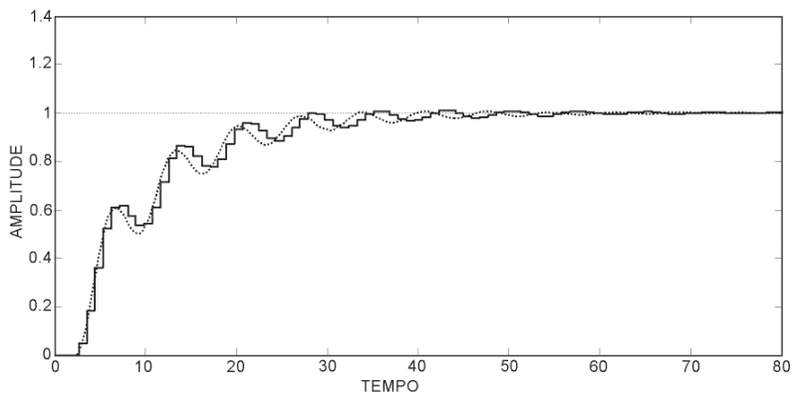# Signals Description (Digital, Analog, Discrete-time, etc)

I really hope you guys can help me. On the picture below we have 2 signals.
Let's call signal X the one that is drawn with a dotted line. The other one we will call signal Y.Now given the 5 options below, where one and only one can be correct, which one would you choose?

B) Signal X is analog in continuous time. Signal Y is discrete.
C) Signal X is discrete. Signal Y is analog in continous time.
D) Signal X is discrete quantized. Signal Y is analog in continous time.
E) Signal X is in continuous time quantized. Signal Y is discrete.

The right answer, according to the people who made the question is (A).
I'm not sure if I agree/understand this, and I hope you guys could open my mind.

The way I see signal X is analog and signal Y is digital.
This is my analysis for each option:
A) Wrong because signal Y is not analog (it has a finity number of possible values for its amplitude) [But it says 'quantized'. Is that ok? To be analog AND quantized?]
B) Could be correct. Y could be a digital version of signal X, so Y is discrete and quantized, meaning a digital signal. How can I know it is not discrete? I now that if it was just an analog discrete signal it would be shown has a sequence of pulses of very low duration, but if it is a digital signal it would be shown just like it is drawn on the image.
C) Can't be correct because signal X is not discrete.
D) Same as C.
E) Can't be correct because signal X is not quantized.

In my opinion this question has answer (B) or none of them. But I could easily been confusing some definitions here (digital, discrete, etc.). I've trying to find those definitions on google but everything was mixed and different from one website to the other. If you guys could give me some help here it would be great. Do you know any books that talk about all of this?

I apologize if the question is too simple for you! And sorry for my english!!

BR.

Bystander
Homework Helper
Gold Member
Check your text and notes for definitions of "discrete" and explicit or implicit initial and final states of zero amplitude for "discrete" signals.

NascentOxygen
Staff Emeritus
I suppose the pair are supposed to be related in some way, but they are not very well drawn to show this.

Obviously, X is continuous. Y resembles a sample-and-hold output (thought it's badly drawn). This means it can take any level, and maintains that level for some time. So I think it can be classified as continuous because there are no quantizing errors in the process. It is analog because it is nowhere represented by a digital value (or at least has none of the associated rounding errors).

I guess 'discrete' means quantized to fixed digital levels. In my view, it is not made clear whether Y can assume any analog level or is restricted to a fixed number of levels. In the absence of obvious digital rounding, I assumed the former, meaning it is continuous.

Bystander
Homework Helper
Gold Member
My suspicion was that "discrete" meant a train of discrete pulses of whatever pulse width is represented, each pulse rising from 0 amplitude, holding at the "quantized" value, then falling again to 0 amplitude --- leaving tracks up and down the plot between pulses. Since no such tracks are evident, "discrete" could be discarded as a possibility. If that isn't part of the definition, then I'm out of ideas.

So you guys agree with (A) being the correct answer? Or do you think there's no correct option at all?

Discretization in this case obviously means dicretization by time interval. Or maybe, this is a quantization. Who would remember that...

Last edited:
donpacino
Gold Member
A makes sense. they both exist at all point of time, Y simply looks like an analog signal that had a zero order hold on it.

Basically when you move an analog signal to a digital signal you will sample it at various points. Lets say you want to move it back to the real world. But the real world is analog, you can't just produce a wave (or what this graph is) at a set amplitude at certain times, and have it not exist at others. You need to decide what to do between the time intervals. The best way to make this real signal is a zero order hold, simply hold the current value until the next time interval, and repeat.

donpacino, I TOTALLY agree with you, signal Y probably is signal X after ZOH. But ZOH does not quantize the signal it just holds it. When option (A) says the signal is analog AND quantized it is wrong. It shouldn't say quantized or it shouldn't say analog.

Analog signal = can have infinity number of values for its amplitude.
Quantized signal = has a finite number of values for its amplitude.

Just remember that the process of quantization does that: approximates the real value of the signal to the closest value for the quantization process.

So, I believe the question has no valid answer!

NascentOxygen
Staff Emeritus